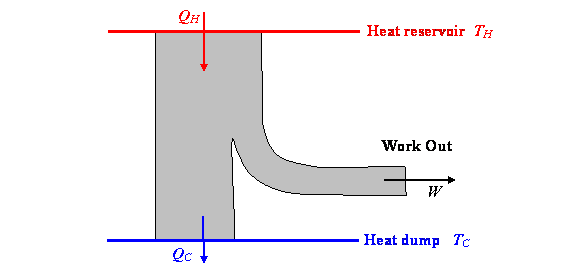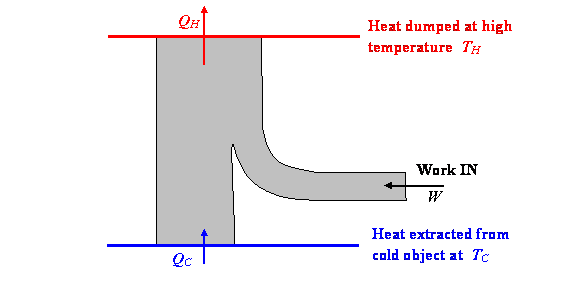$$\require{cancel}$$

# 1.8: The Laws of Thermodynamics and Limits on Engine Efficiency

•• Contributed by Michael Fowler
• Beams Professor (Physics) at University of Virginia

# The Laws of Thermodynamics

(Picture below from St. Andrews)In 1850, Rudolph Clausius, a German physicist, created the science of thermodynamics. He was convinced by Joule’s experiments (he didn’t know about Mayer’s work at the time) that total energy, including kinetic, potential, electrical, and, importantly, heat, was conserved, he called this:

The first law of thermodynamics: total energy, including heat energy, is always conserved.

He explicitly assumed that heat was just the kinetic energy of the moving particles that made up a body. He was the first to make clear that Carnot’s analysis of the heat engine (the cycle) was almost right, that once it is realized that heat is not a conserved fluid, the heat entering the cold reservoir in a cycle is less than that leaving the hot reservoir by just the amount of work done (in an ideal engine), actually a fairly small correction for the temperature ranges in heat engines at the time.

But he also realized the first law wasn’t the whole story! Some processes that do conserve energy never happen.

The second law of thermodynamics: heat always flows from a warm body to a cooler one, never the other way. An equivalent statement is that we cannot devise an engine, working in a cycle, that causes heat to flow from cold to warm unless we supply outside energy.

Translation: your refrigerator won’t work if it’s not plugged into some power supply, and no-one will ever design one that does.

Unlike the first law, there are many ways of writing the second law: our wording above is a paraphrase of Clausius’ original formulation. Kelvin gave it a more “engineering” look:

It is impossible to devise an engine which, working in a cycle, shall produce no effect other than the extraction of heat from a reservoir and the performance of an equal amount of mechanical work.

Exercise: think about how you might prove these two definitions amount to the same thing.

The second law can also be stated in terms of entropy, a concept we’ll cover in the next lecture.

It’s important to note that the first law of thermodynamics, the conservation of total energy including heat, would not be violated by an engine that powered a ship by extracting heat energy from the surrounding seawater. This second law is saying something new: you can’t do that! And, this second law does not follow from the First by logical deduction—it comes (like the First) from experiment and observation.

# How the Second Law Limits Engine Efficiency

An important consequence of the second law is that no engine can be more efficient than the Carnot cycle. Essentially, this is because a “super efficient” engine, if one existed, could be used to drive a Carnot cycle in reverse, which would pump back to the hot reservoir the heat the super efficient engine dumped in the cold reservoir, and the net effect of the two coupled engines would be to take heat from the hot reservoir and do work, contradicting the second law.

To see this, we plot the heat/energy flow for the Carnot cycle:Here $$Q_H = Q_C + W$$ (all expressed in Joules, of course).

Since the engine is reversible, it can also be run backwards (this would be a refrigerator: outside work is supplied, and heat is extracted from a cold reservoir and dumped into a hot reservoir:Suppose now we have a super efficient engine, represented by the first diagram above, and dumping the same heat per cycle QC into the cold reservoir, but taking in more heat energy $$Q_H + \Delta$$ Joules from the hot reservoir, and performing more work: $$W + \Delta$$.

Now, we hook up our super efficient engine to the “Carnot refrigerator” in the other diagram above. The refrigerator sucks out of the cold reservoir all the heat the super efficient engine dumped there, and needs W Joules of work per cycle to do it. The super efficient engine can provide this, and there are still $$\Delta$$ Joules of work to spare. Of course, the Carnot refrigerator has also dumped QH Joules of heat in the hot reservoir. But the bottom line is that between them, the super efficient engine and the Carnot refrigerator have extracted $$\Delta$$ Joules of heat from the hot reservoir and performed $$\Delta$$ Joules of work—contradicting the second law.

The second law therefore forces the conclusion that no amount of machine design will produce an engine more efficient than the Carnot cycle. The rather low ultimate efficiencies this dictated came as a shock to nineteenth century engineers.

Exercise: prove that it is impossible to construct a reversible engine, operating between two temperatures, that is less efficient than the Carnot cycle. (Hint: hook it to a Carnot cycle, drive one backwards.) It follows that although we used an ideal gas in our analysis, that was unnecessary—the same efficiency would result with any reversible engine.